Importance: High ✭✭✭
 Author(s):
 Subject: Geometry
 Keywords: cube decomposition simplex
 Posted by: mdevos on: August 6th, 2008
Question   What is the minimum cardinality of a decomposition of the-cube into-simplices?

A decomposition of a polytope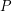into-simplices is a set of-simplices which have pairwise disjoint interiors and have union equal to. This is also known as a (generalized) triangulation.

Letbe the minimum cardinality of a decomposition of the-cube into-simplices (the answer to our question). It is trivial that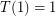and easy to see that. A 3-dimensional cube may be decomposed into five simplices by cutting off every other corner as shown in the figure (from [JW]). This division is optimal, so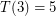.Chopping off every other corner of a 4-cube leaves a 16-cell (the 4-dimensional cross-polytope) which can then be decomposed into eight simplices (fix a vertexand then take each of the eight 4-simplices formed as the convex hull ofand a facet which is not incident with). This is also optimal, so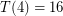. Computer assisted searches have yielded other good decompositions in low dimensions (see [S]).

The decompositions of the 3 and 4-dimensional cubes described here do not generalize to higher dimensions. However, there is a naive decomposition of an-cube into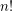simplices. Take the cube to beand letbe the set of all pointsfor which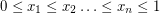. Thenis a simplex contained in our cube which contains the main diagonal from the origin to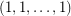. Further, by permuting the terms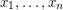in the chain of inequlities, we get a total ofsimplices which form a decomposition of the cube.

This naive decomposition is not optimal in dimensions 3 and 4 since our constructions showand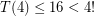. Haiman [H] found a clever way to lift efficient lower dimensional decompositions to high dimensions thus achieving a significant improvement on ourupper bound. To state his result precisely, we require another parameter. Let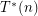be the minimum cardinality of a decomposition of an-cube into-simplices with the following additional constraints:

\item Every vertex of a simplex is a vertex of the cube. \item The intersection of any two simplices is a face of both of them.

It is immediate that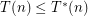, but to the best of our knowledge these parameters may always be identical. Indeed, this is a separate interesting question. Anyway, back to Haiman's bound. He proved that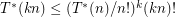. Using this inequality with either the 3 or 4-dimensional example from above would give an improvement on theupper bound. However, best known is to plug in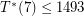, which gives a general upper bound of.

A natural lower bound oncan be obtained by a volume argument. Clearly,must be at least the volume of an-dimensional cube divided by the volume of the largest simplex it contains. Smith [S] improved upon this by moving the argument to hyperbolic space (where the volume of a cube is comparatively much larger than that of a simplex). His volume estimate here yields.

## Bibliography

[H] M. Haiman, A simple and relatively efficient triangulation of the n-cube, Discr. Comp. Geom. 6, 4 (1991) 287-289.

[JW] Jackson, Frank and Weisstein, Eric W. "Tetrahedron." From MathWorld--A Wolfram Web Resource.

[S] W. Smith, A lower bound for the simplexity of the n-cube via hyperbolic volume.

* indicates original appearance(s) of problem.